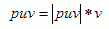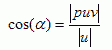Prev: Dot Product Next: Line and Rays

Consider two vectors v and u. The purpose of this section is to show how to compute the projection of vector u onto vector v.The vector puv is the projection of vector u on vector v.

As v and puv share the same direction, and assuming the v is normalized, puv can be defined as:where |puv| stands for the length of puv. So finding out |puv| allows us to easily find vector puv. The relation between the length of u and puv is given by the cosine of the angle between them.The definition of the dot product says thatHence, the value of the length of vector puv is:So, looking back at the first equation, vector puv is defined as:If vector v is normalized, i.e. it has unit length, then the division can be spared.

 Prev: Dot Product Next: Line and Rays

### 2 Responses to “Vector Projection”

1.Note that this final formula for puv already assumes that v is normalized. If v is not normalized, then you have to divide by the square of |v|, instead of just |v|. So, if v is not normalized, you need to use the formula:

puv = ( Dot(v,u) / (|v|^2) ) * v

2.send me worked examples

This site uses Akismet to reduce spam. Learn how your comment data is processed.